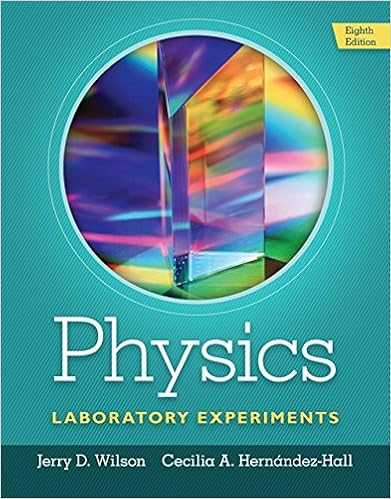# Iv Now consider the reaction: A  B Start with A=B =...

• Test Prep
• 32

This preview shows page 1 - 5 out of 32 pages.

##### We have textbook solutions for you!
The document you are viewing contains questions related to this textbook.The document you are viewing contains questions related to this textbook.
Chapter 9 / Exercise 4
Physics Laboratory Experiments
WilsonExpert Verified
580.221_Exam 3_2016 Name: _____________________________________ 1 Molecules and Cells: Exam 3 Wednesday, December 14, 2016 You are reminded that you are under an honor code at Johns Hopkins and all work on this exam is expected to be entirely your own. You may not use any electronics other than the BME calculators distributed during the exam. You may not use any notes. Read the questions carefully. Most answers require only a very brief explanation. Phrases or bullet points are fine - you do not need to use full sentences. Show all work/calculations for any problems. Clearly indicate the answer to each question. This is a three hour exam so you should have plenty of time to answer each of the questions. This exam has questions from three faculty: Faculty Points Questions/pages Dr. Haase 20 12 questions/6 pages Dr. Yarema 40 3 multi-part questions/ 10 pages Dr. MacGabhann 40 9 questions/14 pages
##### We have textbook solutions for you!
The document you are viewing contains questions related to this textbook.The document you are viewing contains questions related to this textbook.
Chapter 9 / Exercise 4
Physics Laboratory Experiments
WilsonExpert Verified
580.221_Exam 3_2016 Name: _____________________________________ 2 Helpful Equations: Assume R is 1.98 x 10 -3 kcal/(K mole), T is 310 K RT = 0.616 kcal/mol, and F=23.06 kcal volt -1 mol -1 for all problems unless otherwise specified 𝐾𝑒𝑞 = 𝑒 −∆𝐺°/𝑅𝑇 = 10 −∆𝐺°/1.36 eq o K RT G ln ] tan [ ] [ ln ts reac products RT G G o This section for grading: Faculty Points Score Dr. Haase 20 Dr. Yarema 40 Dr. MacGabhann 40 | | | | nFE G m | ] [ ] [ ln | | | in out c X X RT G
580.221_Exam 3_2016 Name: _____________________________________ Dr. Haase: Multiple choice/short answer/short problem 20 pts total 1. (5 pts total, 1 pt each part) Consider the reaction: A i. What is the equilibrium constant , Keq, for this reaction? ii. If the Gibbs free energy of A is less than the Gibbs free energy of B, which of the following is true at equilibrium? (circle all that apply) B
3 iv. Now consider the reaction: A B Start with [A]=[B] = 1M, and [C]=0. Given that the Gibbs free energy of C is less than the Gibbs free energy of B. Will [A] at equilibrium increase or decrease from your answer in part “ii”. Explain: C
iii. Continuing with the situation from above, if [A]=[B] = 1M, which of the following is/are true?
580.221_Exam 3_2016 Name: _____________________________________ v. The Gibbs free energy for a reaction can become negative by:
4
•••